# ISEE Upper Level Math : How to find the perimeter of a rectangle

## Example Questions

### Example Question #1 : How To Find The Perimeter Of A Rectangle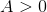. Which is the greater quantity?

(a) The perimeter of the square on the coordinate plane with vertices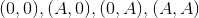(b) The perimeter of the rectangle on the coordinate plane with vertices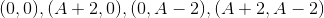It is impossible to tell from the information given.

(a) is greater.

(a) and (b) are equal.

(b) is greater.

(a) and (b) are equal.

Explanation:

(a) The square has sidelength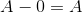, and therefore has perimeter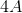.

(b) The rectangle has width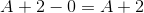and height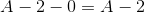, so therefore has perimeter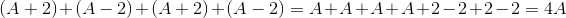.

The perimeters are the same.

### Example Question #2 : How To Find The Perimeter Of A Rectangle

Your geometry book has a rectangular front cover which is 12 inches by 8 inches.

What is the perimeter of your book cover?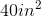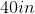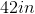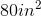Explanation:

Your geometry book has a rectangular front cover which is 12 inches by 8 inches.

What is the perimeter of your book cover?

Perimeter is the distance around the outside of a shape.

Perimeter of a rectangle can be found via the following: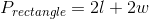Plug in our length and width to find our answer: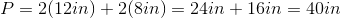### Example Question #3 : How To Find The Perimeter Of A Rectangle

Your aunt and uncle live on a rectangular plot of land out in the countryside. The width of their plot isof a kilometer long. The length is twice the width. What is the total length of the perimeter of the plot of land?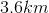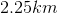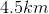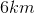Explanation:

Your aunt and uncle live on a rectangular plot of land out in the countryside. The width of their plot isof a kilometer long. The length is twice the width. What is the total length of the perimeter of the plot of land?

Begin by finding both the width and length.

We are told that the width isof a kilometer

Further, we are told that the length is twice the width. Write this as an equation and solve: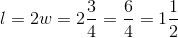Now, find the perimeter via the following: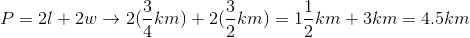So, our perimeter is 4.5 km